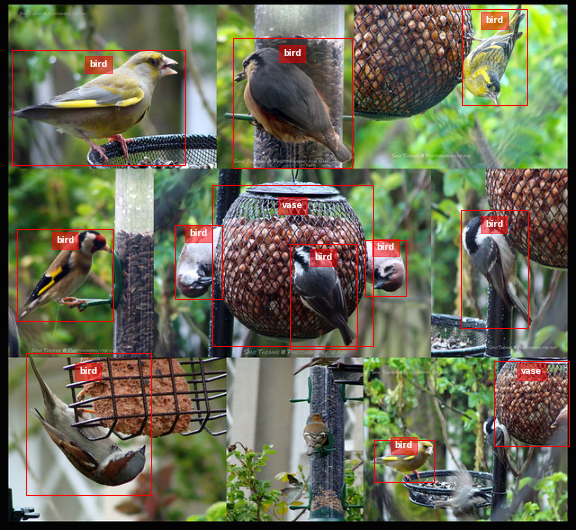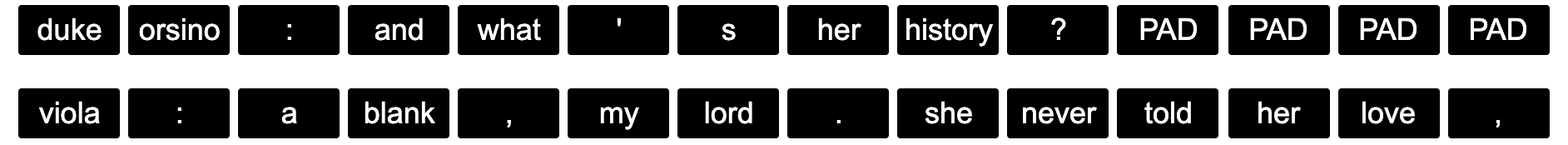### Furthest Point Sampling

I have come across an algorithm named furthest point sampling or sometimes farthest point sampling a few times in deep learning papers dealing with point cloud data. However there is a lack of resources online explaining what this algorithm actually does. Here I will try to explain it with reference to this CUDA implementation. Along the way we will also see how to implement it in an efficient parallelised way.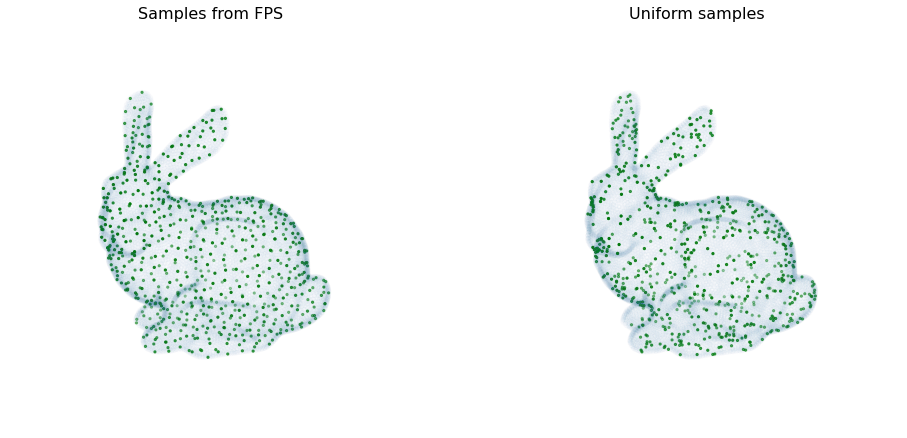## Introduction

In the previous post we motivated the idea of a Variational Auto Encoder. Here we will have a go at implementing a very simple model to get a sense of all the components steps involved.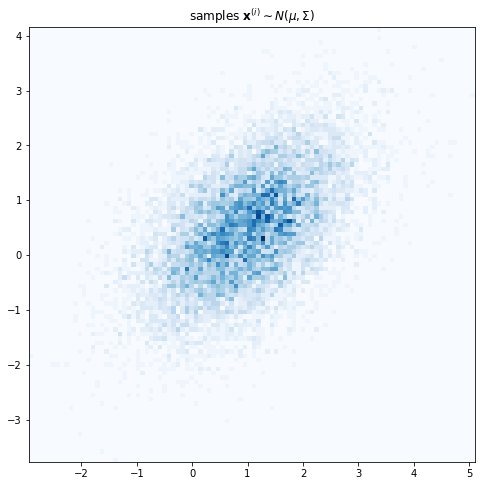### Variational Auto-Encoder - Part 1 / Introduction

Consider this painting by Vincent Van Gogh known as “Wheatfield with Crows”. Suppose you want to learn a probability distribution over Van Gogh’s paintings. What the computer or a deep learning algorithm sees when it looks at this painting is a grid of RGB pixel intensities. So one approach might be to model the data as a sequence of individual pixels. Yet that is manifestly not how the artist has constructed the painting.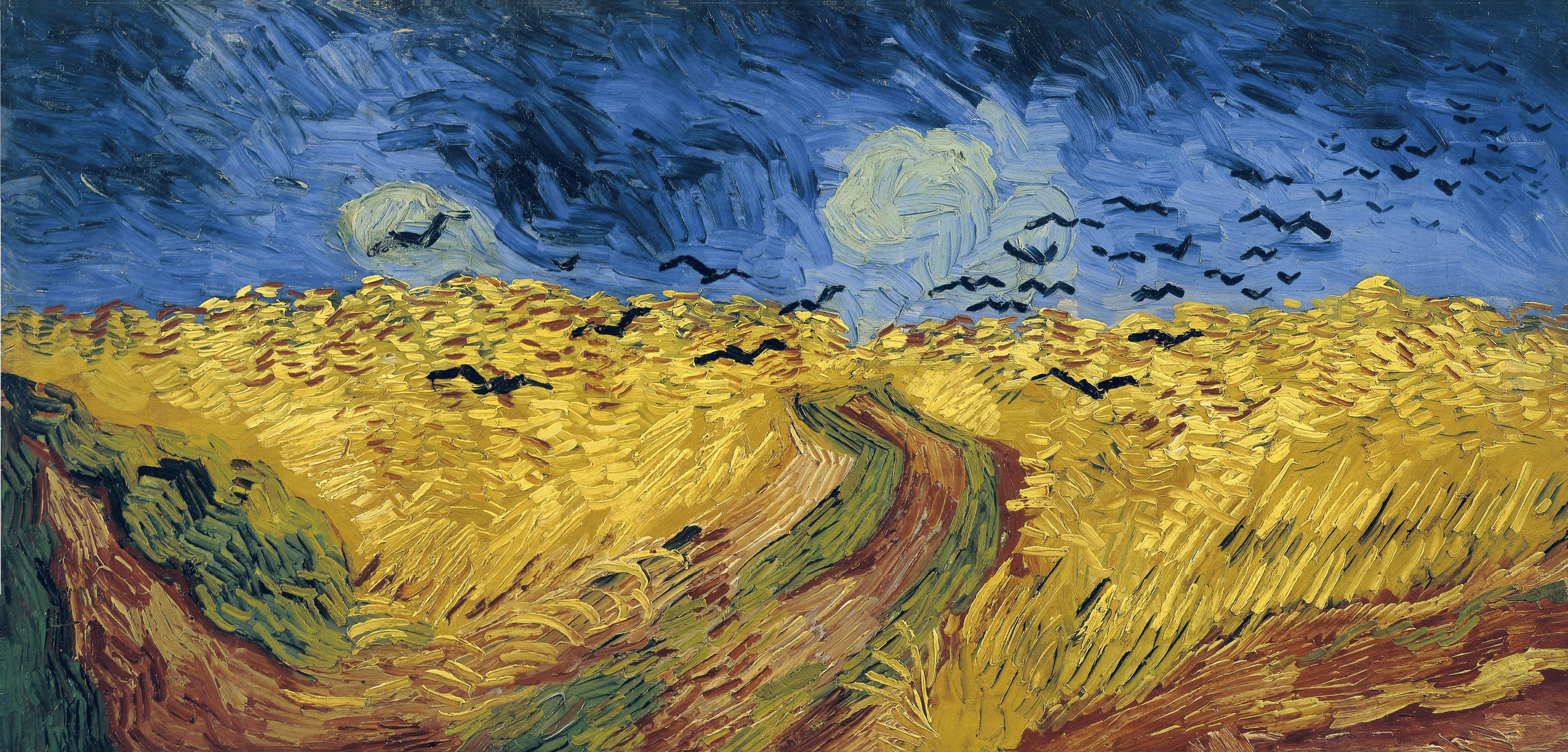### Generating crops from images

This post explains how to implement a function to generate a crop of a particular object from an image given the position of the centroid of the object. The images below show an example use case: cropping all the individual oranges from an image.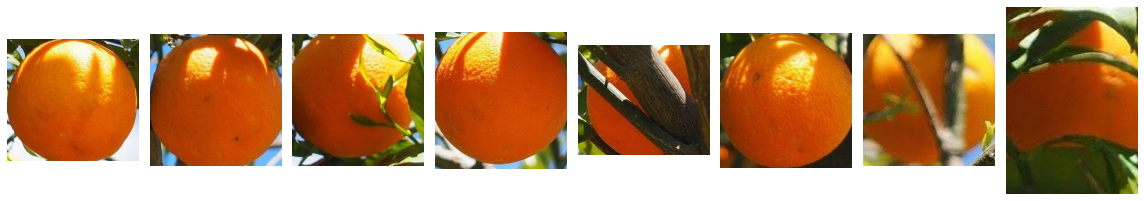### Non-maximum suppression (NMS)

This blogpost contains a quick practical introduction to a popular post-processing clustering algorithm used in object detection models. The code used to generate the figures and the demo can be found in this Colab notebook.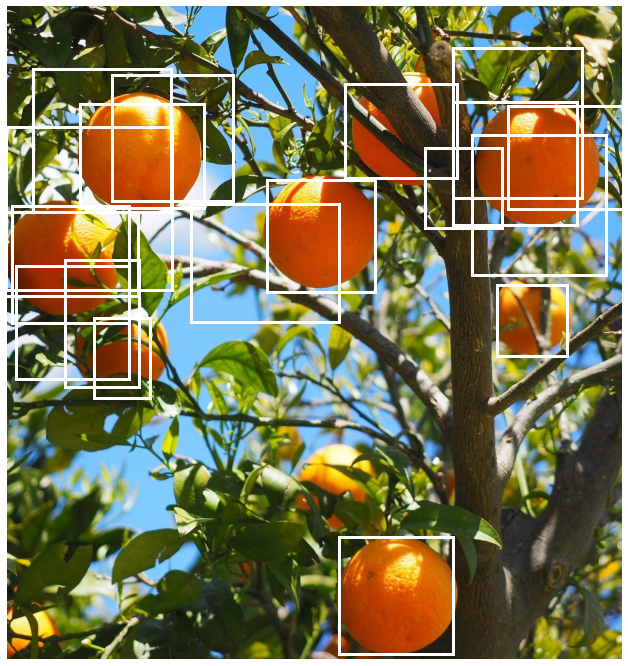### Vectorizing Intersection over Union (IoU)

Supporting batched data is an important requirement for a deep learning pipeline to enable time efficient model training. Often when writing a function, the easiest way to start is to first handle one element and then use a for loop over all the elements in the batch.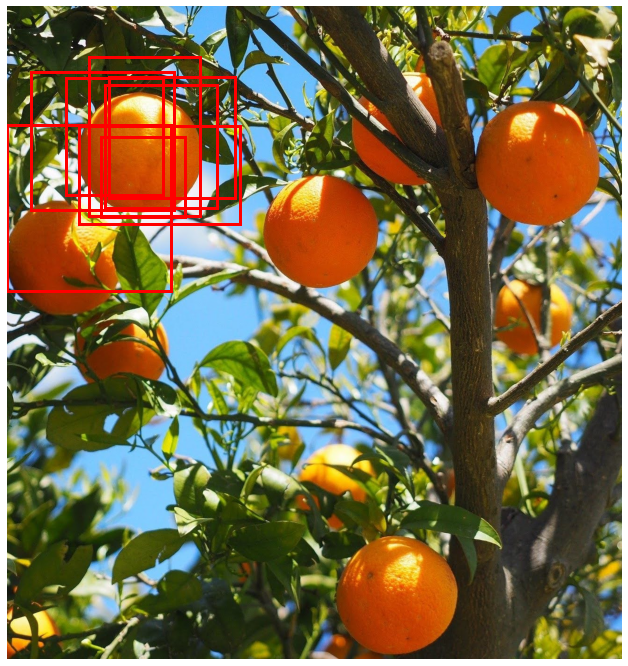### Converting Camera Conventions

When working with computer vision models we frequently need to know information about the camera that was used to generate the image. This includes information about the position of the camera in the world coordinate system as well as the intrinsic properties of the camera such as the focal length.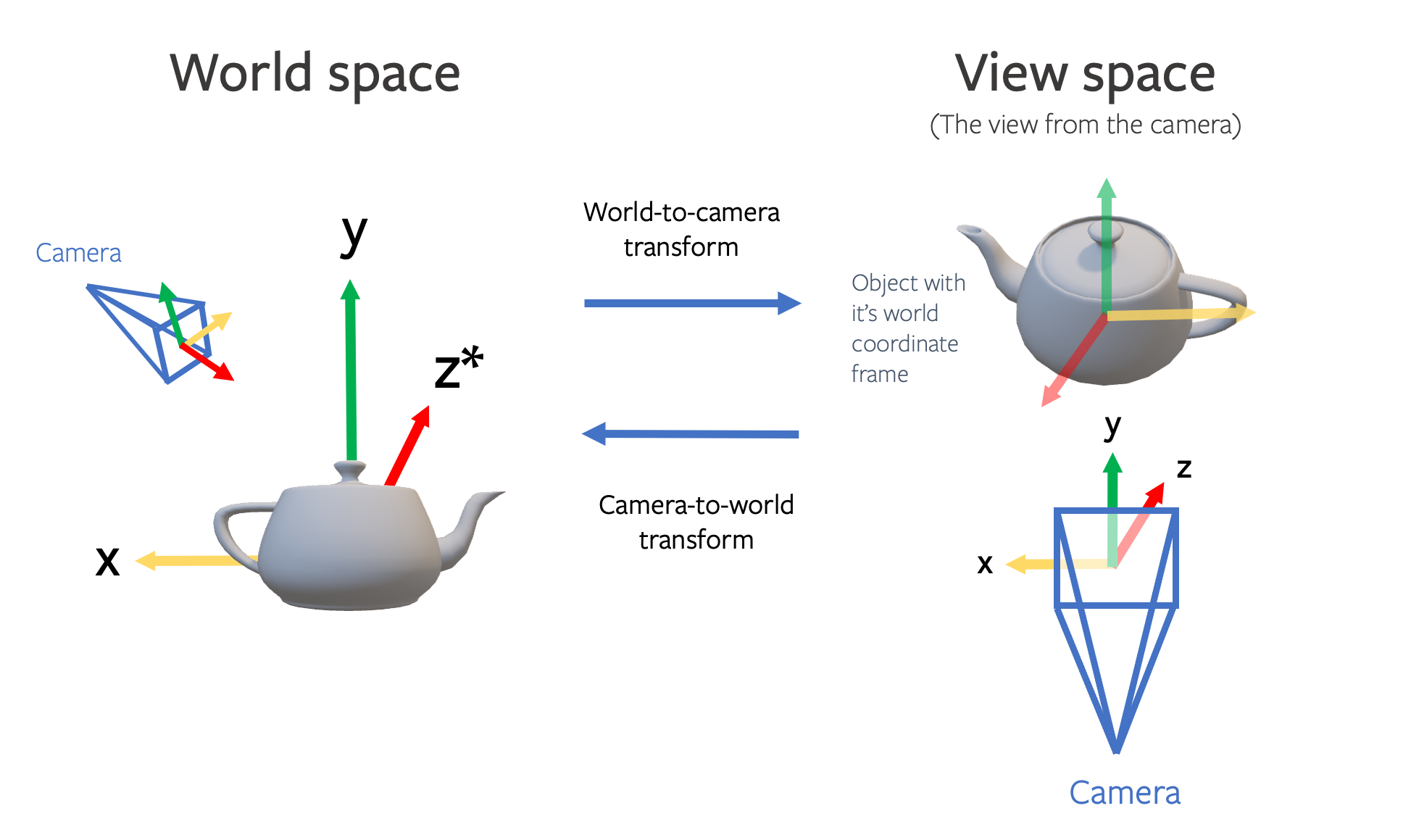### Cosine Learning Rate Decay

In this post we will introduce the key hyperparameters involved in cosine decay and take a look at how the decay part can be achieved in TensorFlow and PyTorch. In a subsequent blog we will look at how to add restarts.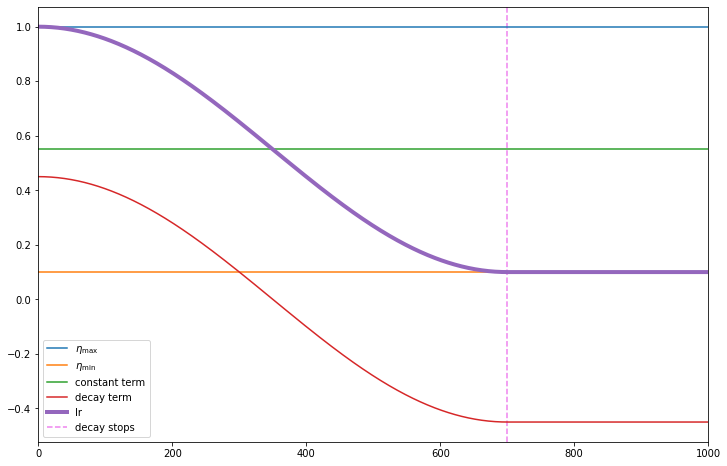### Plotting bounding boxes for object detection

When working with detection models in a pipeline you often need to visualize the bounding boxes to sanity check the predictions. Bounding boxes can be defined in different ways so this is also useful to confirm the convention being used.